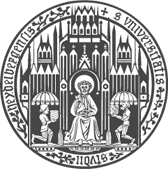### Abstract

We propose the use of the vector-valued distance to compute distances and extract geometric information from the manifold of symmetric positive definite matrices (SPD), and develop gyrovector calculus, constructing analogs of vector space operations in this curved space. We implement these operations and showcase their versatility in the tasks of knowledge graph completion, item recommendation, and question answering. In experiments, the SPD models outperform their equivalents in Euclidean and hyperbolic space. The vector-valued distance allows us to visualize embeddings, showing that the models learn to disentangle representations of positive samples from negative ones.